## MSC (Biotechnology) Final Year Model Papers

#### Top Model Papers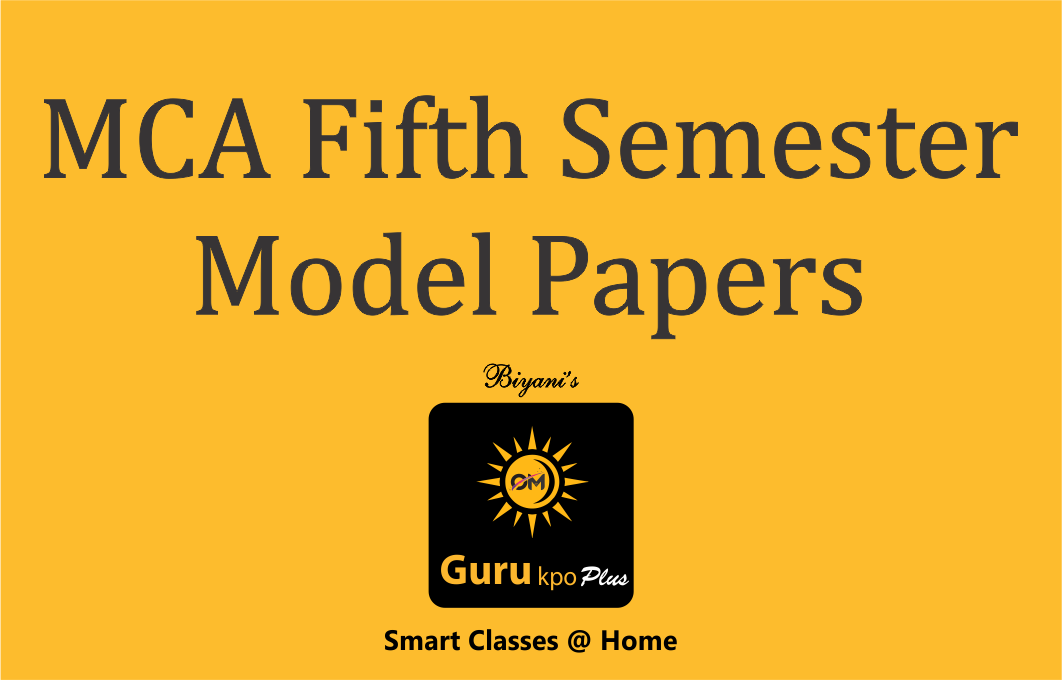#### MCA Fifth Semester Model Papers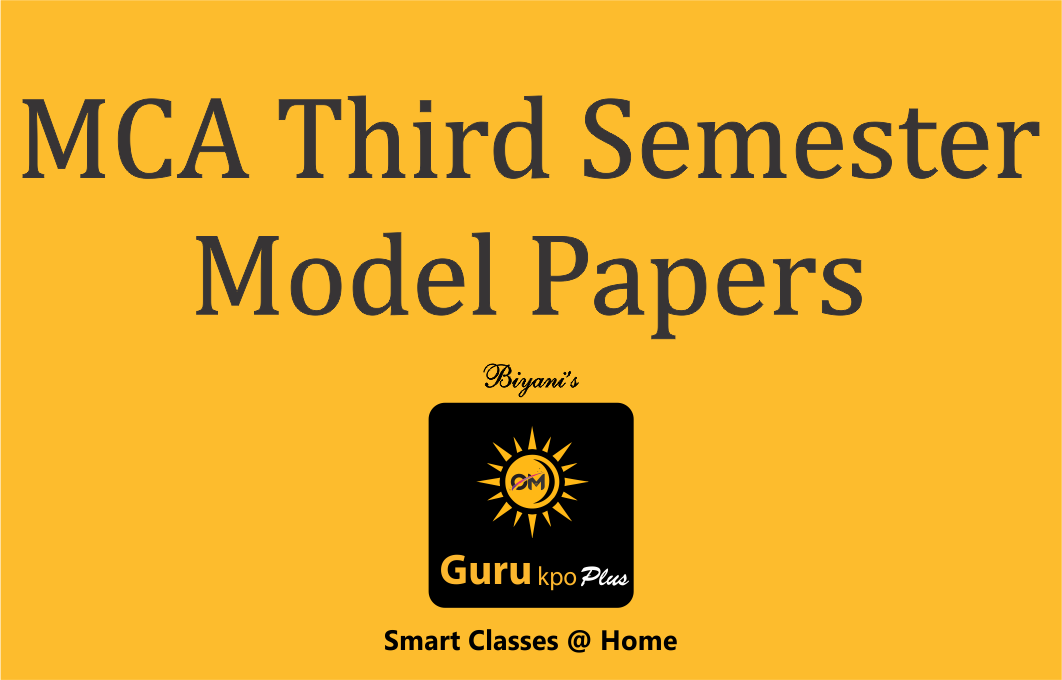#### MCA Third Semester Model Papers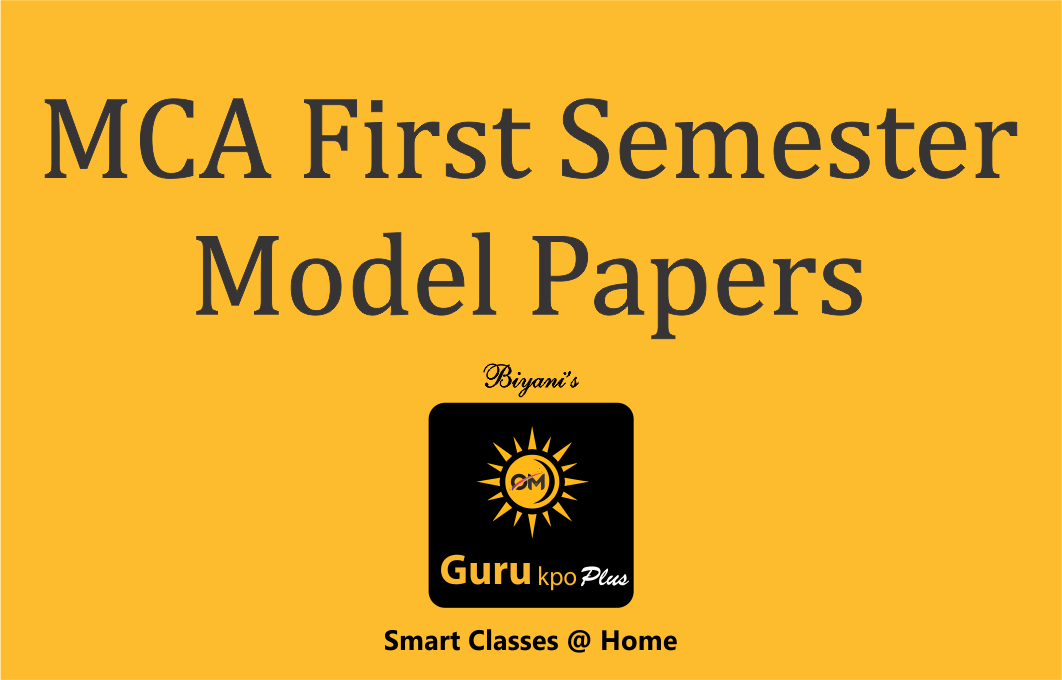#### MCA First Semester Model Papers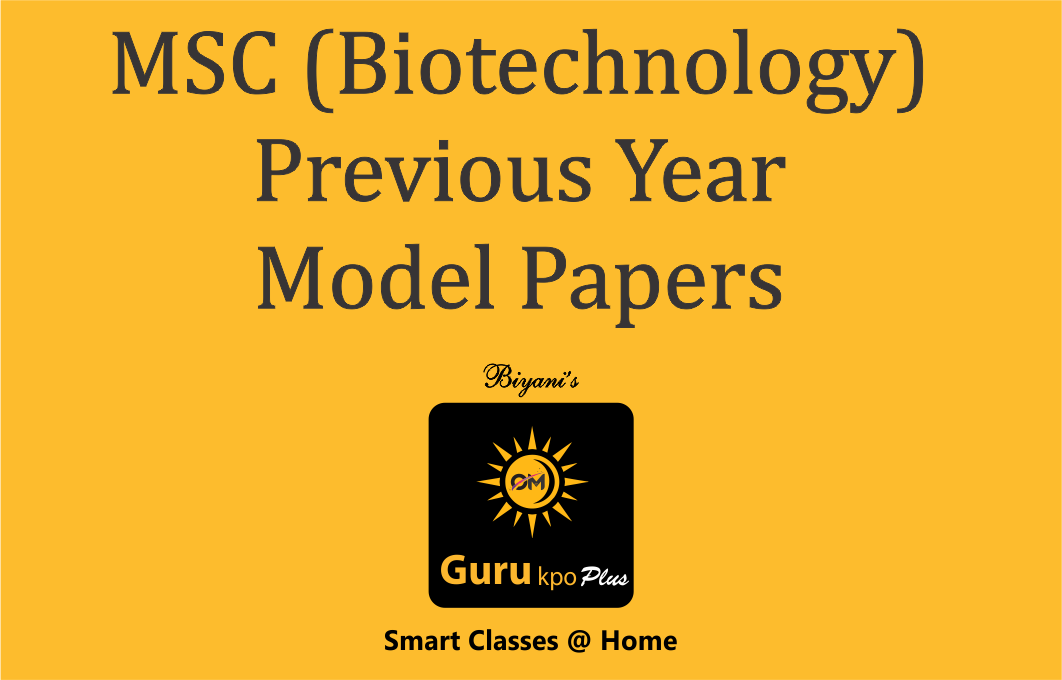#### MSC (Biotechnology) Previous Year Model Papers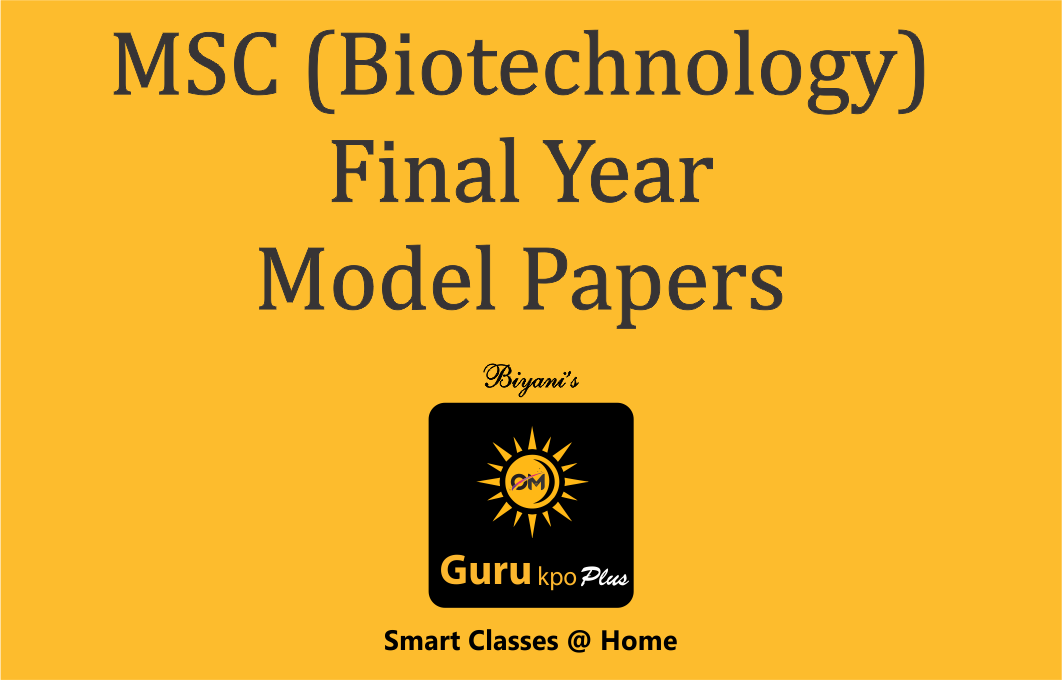#### MSC (Biotechnology) Final Year Model Papers### Equation Of Plane in Three Point Form by Ms. Megha Sharma, Biyani Group of Colleges

In this video MS. MEGHA SHARMA, Biyani Group of Colleges, Jaipur, explains about the Equation of Plane in Three Point Form i.e.( x1,y1,z1), (x2,y2,z2), (x3,y3,z3).Let the equation of plane be-Ax+By+Cz+D =0 …### Integration by Parts (HINDI) B.Sc, M.Sc

In this Video, –if u & v are the function of x then we can write rule of Integration by Parts = (First function )x i(integration of second function )-differential of …### Linear In equation Lecture by Ms. Megha Sharma.

When the two expressions are connected by ‘greater than’ or ‘less than’ sign, we get an inequality. When operating in terms of real numbers, linear inequalities are the ones written in …### Combination Lecture by Miss Priyanka Pandey

This is a part of lecture presented by Miss Priyanka Pandey, Asst. Professor of Biyani Groups of College. The video is about combination. The ways of selecting object or persons from …### Permutation (B.Sc, MSc)

This is a part of lecture presented by Miss Priyanka pandey ,Asst. professor of Biyani Groups of College. The video is about permutation. Permutation used for order of selection i.e. order …### Area Between Two Cartesian Curves-Functuions (B.Sc Maths)

Dr. Radhika Goyal, Assistant Professor, Biyani Group of Colleges, describes about area between two Cartesian curves. If two curves, y1 = f1 (x) and y2 = f2 (x) are given then …### Differentiation, Gurukpo

Differentiation, Gurukpo